## 7.14Thermal wall functions

Wall functions were introduced in Sec. 7.5 in order to improve the calculation of wall shear stress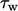when cells are too large near a wall to resolve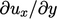accurately. The same problem exists with heat ﬂux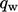and an under-predicted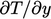. As before, the universal character of the boundary layer can be exploited, this time to improve the calculation of.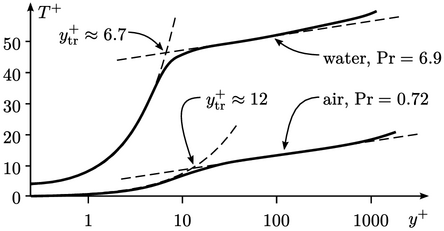The temperature distribution is characterised by Eq. (7.51 ) for the viscous sub-layer, and the log law Eq. (7.52 ) for the inertial sub-layer. The transition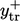for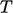occurs at the intersection of the two equations, i.e. when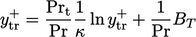(7.57)
While the transition between these regimes is ﬁxed at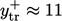by Eq. (7.18 ) for the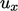proﬁle, the (iterative) solution of Eq. (7.57 ) is dependent on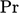and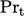.

Using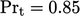and Eq. (7.55 ) for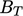,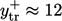for air at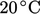with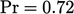. For water under the same conditions,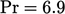and the corresponding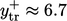.

A wall function can be derived which adjusts the turbulent conductivity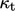, in a similar manner to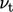in the standard wall function in Sec. 7.5 . The model calculates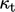for each patch face based on the near-wall cell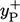.

No adjustment is made to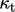when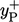corresponds to the viscous sub-layer. Whencorresponds to the inertial sub-layer,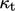is calculated as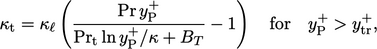(7.58)
where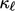denotes the laminar thermal conductivity, i.e.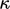from Eq. (2.54 ), to distinguish it from Kármán’s constant. Eq. (7.58 ) usesfrom Eq. (7.19 ), as in the standard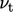wall function.

The wall function is derived based on adjusting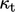to improve the numerical calculation of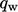by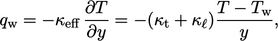(7.59)
where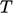represents a value close to the wall, e.g. in a near-wall cell. By comparison, Eq. (7.49 ) and Eq. (7.50 ) combine to give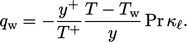(7.60)
The heat ﬂux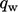is consistent between Eq. (7.59 ) and Eq. (7.60 ) when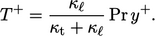(7.61)
When the log law Eq. (7.52 ) is then substituted in Eq. (7.61 ), it provides the thermal wall function model which adjustsaccording to Eq. (7.58 ).
Notes on CFD: General Principles - 7.14 Thermal wall functions